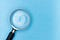# Modified Binary Search Algorithm to Solve Tricky Problems

## Solve problems by modifying the Binary Search Algorithm

Binary Search is the most popular Searching Algorithm which is most asked in coding interviews. Its popularity is because of it’s time complexity, where the linear search algorithm takes O(N) time, the Binary Search takes O(log N) time. The only condition in Binary Search is that the array should be sorted.Photo by Markus Winkler on Unsplash

In this article, I am going to solve a tricky problem of Binary Search asked in LeetCode called “Find the minimum element in a Rotated and Sorted array” or “Find the Pivot Element in the Rotated and Sorted array”

This is a tricky problem because it cannot be solved by the standard Binary Search Algorithm since the array is rotated.

# Problem Statement:

`Suppose an array sorted in ascending order is rotated at some pivot unknown to you beforehand.(i.e.,  [0,1,2,4,5,6,7] might become  [4,5,6,7,0,1,2]).Find the minimum element.Input: [3,4,5,1,2] Output: 1`

# Problem’s Approach:

The reason why Binary Search provides O(log N) time complexity is that on each iteration it eliminates half of the array and searches the element in the remaining half.

We need to implement the same approach here and find a way to eliminate half of the array in each iteration.

# Problem’s Solution:

Let us look at this rotated array example carefully:

`Example: [4,5,6,7,0,1,2] // minimum element 0 at index 5`

By observing the array we can conclude two points:

1. The index of the minimum element is the same as the number of rotations of the array
2. The minimum element is smaller than its adjacents

But How do we eliminate one half of the array through Binary Search?

The approach is similar to the standard binary search where we find the middle element of the array but the condition changes. Hence by modifying the standard Binary Search Algorithm we get the solution.

When we find the middle element ( in the above example number 7 is the middle element ) we can observe that the array is divided into two halves where one half is sorted and the other half is unsorted. Another interesting observation is that the minimum element is always in the unsorted half.

Algorithm:

1. Find the middle element of the array.
2. Check if the middle element is the minimum element
3. If the middle element does not satisfy the minimum element condition then apply binary search on the unsorted half of the array.

Code Implementation in Javascript:

Step 1: Set left and right values. In this problem, the left value is 0 and the right value is the length of the array.

`let left = 0;let right = nums.length - 1;`

Step 2: Find the middle element of the array and check for the unsorted half of the array.

`const mid = Math.floor((left + right) / 2);if (arr[mid] > arr[right]) {   left = mid + 1;} else {   right = mid;}`

When the middle value is greater than the rightmost value of the array, it shows that the right half of the array is unsorted, or else the left side is unsorted.

Step 3: Return the answer. The loop breaks when the left is greater than or equal to the right value. This happens when we have found the minimum element in the array as both left and right are equal we return the left index.

`return arr[left];`

# Final Solution:

Thank you for reading the article till the end, hope this article will help you in your coding preparations. All the best!!!

Self-taught Developer | Tech & Self Help Blogger | Exploring Life and Tech | Aim to Help Upcoming Software Developers in their Journey

## More from Ashutosh Kumar

Self-taught Developer | Tech & Self Help Blogger | Exploring Life and Tech | Aim to Help Upcoming Software Developers in their Journey